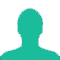# "BAUD" rate means

A. The number of bits transmitted per unit time.

B. The number of bytes transmitted per unit time.

C. The rate at which the signal changes.

D. None of the above

1.The baud rate is the rate at which information is transferred in a communication channel. In the serial port context, "9600 baud" means that the serial port is capable of transferring a maximum of 9600 bits per second.

At baud rates above 76,800, the cable length will need to be reduced. The higher the baud rate, the more sensitive the cable becomes to the quality of installation, such as how much of the wire is untwisted around each device.
It is quiet similar to Bit rate but here are the differences:
Bit rate is the transmission of number of bits per second. On the other hand, Baud rate is defined as the number of signal units per second. The formula which relates both bit rate and baud rate is given below:

Bit rate = Baud rate x the number of bit per baud.
Let’s see the difference between Bit Rate and Baud Rate:

S.NO BIT RATE BAUD RATE
1. Bit rate is defined as the transmission of number of bits per second. Baud rate is defined as the number of signal units per second.
2. Bit rate is also defined as per second travel number of bits. Baud rate is also defined as per second number of changes in signal.
3. Bit rate emphasized on computer efficiency. While baud rate emphasized on data transmission.
4. The formula of Bit Rate is:
= baud rate x the number of bit per baud
The formula of Baud Rate is:
= bit rate / the number of bit per baud

2.What is BAUD ?

Related Questions on Networking Miscellaneous

A WATS arrangement

A. is always less expensive than flat-rate service

B. is less expensive than flat-rate service only when the number of calls is large and the duration of each is short

C. is less expensive than flat-rate service only when the number of calls is small and the duration of each is long

D. is never less expensive than flat-rate service

E. None of the above